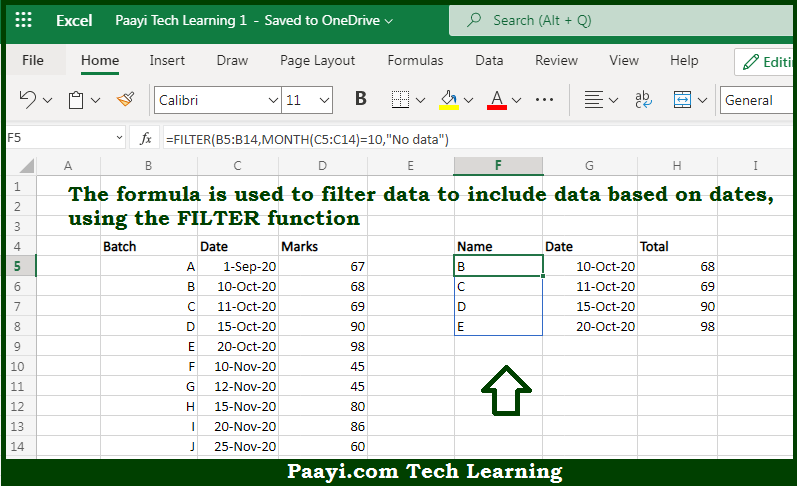# Learn How to Filter By Date in Microsoft Excel

Written by | 0 Comments | 601 Views

In this article, you will learn how to evaluate things in Dynamic Arrays with formulas in Microsoft Excel using a single/combination(s) of functions. You will also know How to Filter By Date and see the generic formula.

Learn How to Filter By Date in Microsoft Excel

The main purpose of this formula is to filter data to include data based on dates. Here we will learn how to filter by the date the given data range in the workbook in Microsoft Excel. That implies, with the help of formula based on the FILTER function you can able to filter data to include data based on dates. So, with the help of this formula, you can able to filter by the date the given data range in the workbook in Microsoft Excel.

General Formula to Filter By Date

=FILTER(rng1,MONTH(rng2)=7,"no data")

The Explanation for the Filter By DateSo we know that with the help of the given formula above you can able to filter data to include data based on dates. Here we will learn how to filter by the date the given data range in the workbook in Microsoft Excel. As we know that here the formula relies on the FILTER function to retrieve data based on a logical test created with the MONTH function. The array argument is provided as the named range "data", which contains the full set of data without headers. It should be noted that the argument is constructed with the MONTH function. So, with the help of this formula, you can able to filter data to include data based on dates. Here we will learn how to filter by the date the given data range in the workbook in Microsoft Excel.